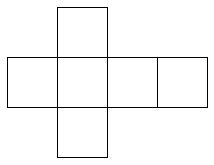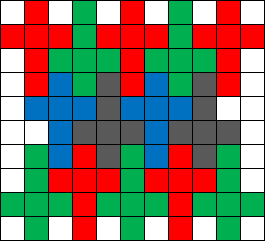# Strange tiles

Consider a $10 \times 11$ rectangular room which is divided into $110$ unit squares in the natural way by lines parallel to the sides of the room. We also have a collection of strange tiles. Each tile consists of six unit squares connected together in the following shape:What is the maximal possible number of non-overlapping tiles we can pack into the room such that each tile is covering exactly six unit squares of the room? Give proof and diagram of the tiling.Note by Sharky Kesa
5 years, 9 months ago

This discussion board is a place to discuss our Daily Challenges and the math and science related to those challenges. Explanations are more than just a solution — they should explain the steps and thinking strategies that you used to obtain the solution. Comments should further the discussion of math and science.

When posting on Brilliant:

• Use the emojis to react to an explanation, whether you're congratulating a job well done , or just really confused .
• Ask specific questions about the challenge or the steps in somebody's explanation. Well-posed questions can add a lot to the discussion, but posting "I don't understand!" doesn't help anyone.
• Try to contribute something new to the discussion, whether it is an extension, generalization or other idea related to the challenge.

MarkdownAppears as
*italics* or _italics_ italics
**bold** or __bold__ bold
- bulleted- list
• bulleted
• list
1. numbered2. list
1. numbered
2. list
Note: you must add a full line of space before and after lists for them to show up correctly
paragraph 1paragraph 2

paragraph 1

paragraph 2

[example link](https://brilliant.org)example link
> This is a quote
This is a quote
    # I indented these lines
# 4 spaces, and now they show
# up as a code block.

print "hello world"
# I indented these lines
# 4 spaces, and now they show
# up as a code block.

print "hello world"
MathAppears as
Remember to wrap math in $$ ... $$ or $ ... $ to ensure proper formatting.
2 \times 3 $2 \times 3$
2^{34} $2^{34}$
a_{i-1} $a_{i-1}$
\frac{2}{3} $\frac{2}{3}$
\sqrt{2} $\sqrt{2}$
\sum_{i=1}^3 $\sum_{i=1}^3$
\sin \theta $\sin \theta$
\boxed{123} $\boxed{123}$

Sort by:

It is easy to pack 14:I can also prove that this number is at most 15: the corner squares can't be used, and if a square on the edge is used then the two adjacent squares on the edge aren't used either, which totals to $2 \times 5 = 10$ squares from the long sides, $2 \times 4 = 8$ squares from the short side, and $8 \times 9 = 72$ squares from the middle, for a total of $10+8+72 = 90$ squares or $\left\lfloor \frac{90}{6} \right\rfloor = 15$ pieces.

Since the packing for 14 looks solid, I believe there shouldn't be any packing for 15, but I haven't proved it.

- 5 years, 8 months ago

I think you can lower the upper bound from 15 to 14 by looking at the squares adjacent to the corners.

If both of them are filled, then on at least one side, at least two squares will be empty. This should bring down the number of usable squares to $88$.

- 5 years, 8 months ago

My guess is 13, but I have no proof it is the best

- 5 years, 9 months ago

Do you have a diagram at the very least?

- 5 years, 9 months ago- 5 years, 9 months ago